## How To Copy Only Visible Cells In Google Sheets

In this post, you’ll learn how to copy only visible cells in Google Sheets so that you only paste the results you want and don’t include any hidden data.

For this tutorial, we’ll use this dataset, shown in full to begin: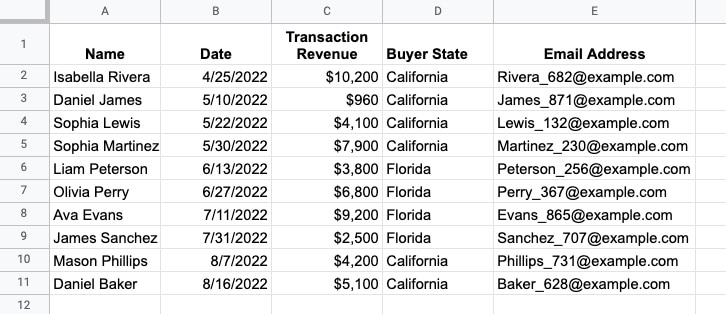Now suppose that columns B and C, and rows 6 – 9, are hidden so the data looks like this: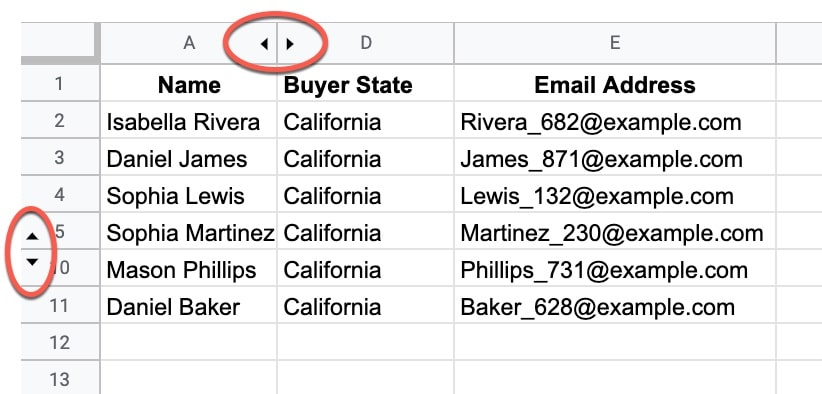If you just highlight this data and copy it, then when you paste it elsewhere the hidden data shows up.

So, how do you copy only visible cells?

## How To Draw The Sierpiński Triangle In Google Sheets

The Sierpiński triangle is a fractal set in the shape of an equilateral triangle, divided into smaller triangles infinitely.

Graphically, we can draw an approximation of the Sierpiński triangle in Google Sheets: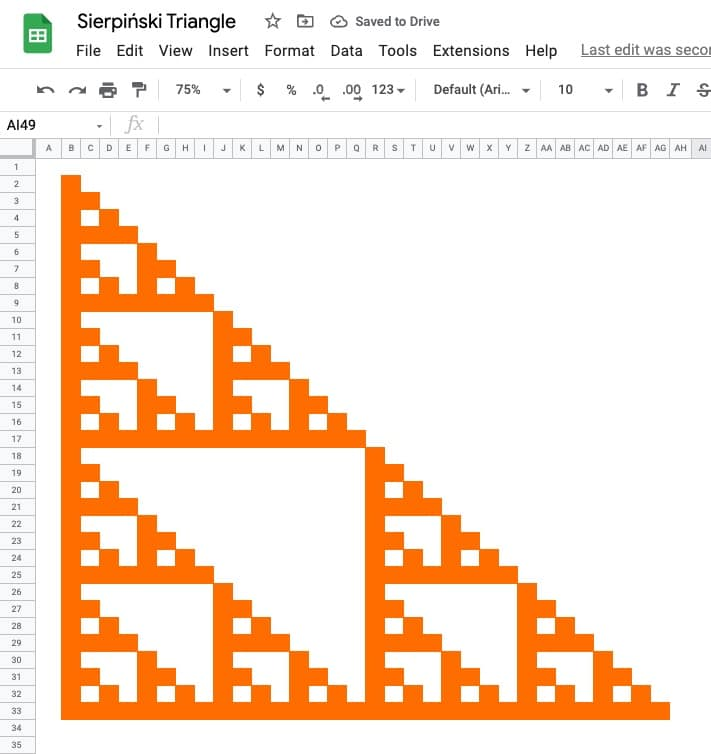🔗 Get this example and others in the template at the bottom of this article.

It is named after the Polish mathematician Wacław Sierpiński and is also known as the Sierpiński gasket or Sierpiński sieve.

It has the property of being self-similar, meaning it looks the same at any magnification.

See Wikipedia for more on the Sierpiński triangle.

## The LARGE Function In Google Sheets

The LARGE function in Google Sheets returns the n-th largest value from a dataset.

For example, you could use large to determine the 5th largest value in a dataset, the 10th largest, the 50th largest, etc.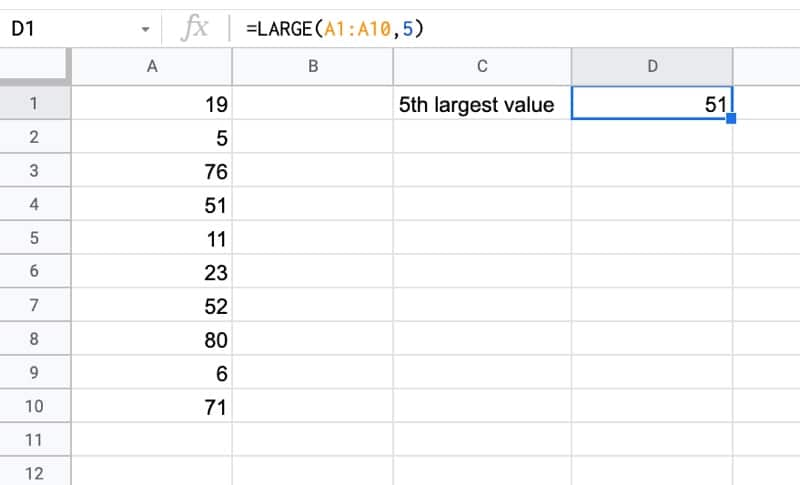The formula to find the 5th largest value in this example is:

`=LARGE(A1:A10,5)`

🔗 Get this example and others in the template at the bottom of this article.

## SORTN Function In Google Sheets

The SORTN function in Google Sheets sorts your data and returns the first n results.

One common use case is to find the top n or the bottom n values in a dataset.

For example, here’s how you use SORTN to extract the top 5 values in a dataset: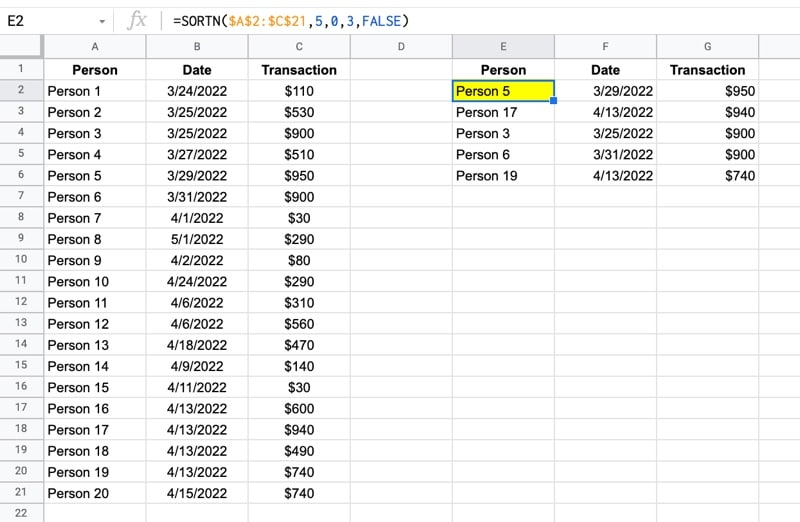with this formula:

`=SORTN(A2:C21,5,0,3,FALSE)`

🔗 Get this example and others in the template at the bottom of this article.

## How To Highlight The Top 5 Values In Google Sheets With Formulas

In this post, you’ll learn how to find and highlight the top 5 values in Google Sheets.

For all the examples that follow, we’ll use this dataset, which is available in the downloadable template at the end of this post: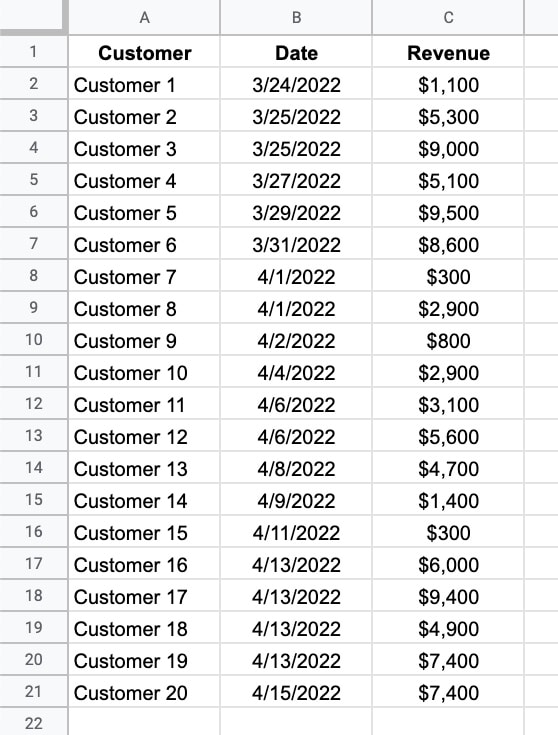We’ll see how to highlight the rows with the top 5 values, as well as how to extract those values using SORTN.